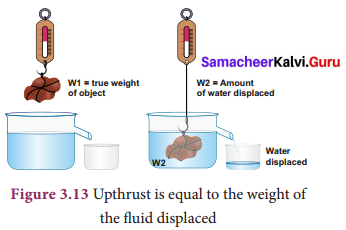# Samacheer Kalvi 9th Science Solutions Chapter 3 Fluids

## Tamilnadu Samacheer Kalvi 9th Science Solutions Chapter 3 Fluids

### Samacheer Kalvi 9th Science Fluids Textbook Exercises

Chapter 3 Fluids Question 1.
The size of an air bubble rising up in water ……………. .
(a) decreases
(b) increases
(c) remains same
(d) may increase or decrease
(a) decreases

Fluids 9th Science Question 2.
Clouds float in atmosphere because of their low ……………. .
(a) density
(b) pressure
(c) velocity
(d) mass
(a) density

9th Science Fluids Question 3.
In a pressure cooker, the food is cooked faster because ……………. .
(a) increased pressure lowers the boiling point
(b) increased pressure raises the boiling point
(c) decreased pressure raises the boiling point
(d) increased pressure lowers the melting point
(a) increased pressure lowers the boiling point

Fluids Lesson 9th Class Question 4.
An empty plastic bottle closed with an airtight stopper is pushed down into a bucket filled
with water. As the bottle is pushed down, there is an increasing force on the bottom as shown in graph. This is because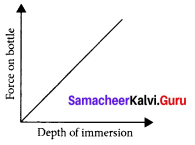(a) more volume of liquid is displaced
(b) more weight of liquid is displaced
(c) pressure increases with depth
(d) all the above
(d) all the above

II. Fill in the blanks.

1.  The weight of the body immersed in a liquid appears to be ………….. than its actual weight
2.  The instrument used to measure atmospheric pressure is …………….. .
3.  The magnitude of buoyant force acting on an object immersed in a liquid depends on ………….. of the liquid.
4.  A drinking straw works on the existence of …………….. .

1.  less
2.  barometer
3.  density
4. Pressure

III. True or False.

Samacheerkalvi.Guru 9th Science Chapter 3 Fluids Question 1.
The weight of fluid displaced determines the buoyant force on an object.
True.

Samacheer Kalvi.Guru 9th Science Chapter 3 Fluids Question 2.
The shape of an object helps to determine whether the object will float.
True.

Samacheer Kalvi Guru 9th Science Guide Chapter 3 Fluids Question 3.
The foundations of high-rise buildings are kept wide so that they may exert more pressure on the ground.
True.

Samacheer Kalvi Guru 9th Science Guide Chapter 3 Fluids Question 4.
Archimedes’ principle can also be applied to gases.
False.
Correct statement: Archimedes’ principle is about fluid displacement which does not involve gases.

Class 9 Science Chapter 3 Question Answer Question 5.
Hydraulic press is used in the extraction of oil from oil seeds.
True.

IV. Match the following.

 Column – I Column – II (a) Density (i) hpg (b) 1 gwt (ii) Milk (c) Pascal’s law (iii) $$\frac { Mass }{ Volume }$$ (d) Pressure exerted by a fluid (iv) Pressure (e) Lactometer (v) 980 dyne

(a) (iii)
(b) (v)
(c) (iv)
(d) (i)
(e) (ii)

Samacheer Kalvi Guru Science 9th Question 1.
On what factors the pressure exerted by the liquid depends on?
Pressure exerted by a liquid at a point is determined by,

•  depth (h)
•  density of the liquid (p)
•  acceleration due to gravity (g).

Class 9 Science Chapter 3 Solutions Question 2.
Why does a helium balloon float in air?
Helium is much less denser than ordinary air which gives them buoyancy and thus float in air.

Science Class 9 Chapter 3 Question Answer Question 3.
Why it is easy to swim in river water than in sea water?
Due to the presence of dissolved salts in sea water is denser than river water which makes floating easier and hence swimming is easier.

Science Chapter 3 Exercise Question 4.
What is meant by atmospheric pressure?
The pressure exerted by the atmospheric gases on its surroundings and on the surface of the earth is called atmospheric pressure.

Class 9 Science Chapter 3 Solution Question 5.
State Pascal’s law.
Pascal’s law states that an increase in pressure at any point inside a liquid at rest is transmitted equally and without any change, in all directions to every other point in the liquid.

Class 9th Science Chapter 3 Question 1.
With an appropriate illustration prove that the force acting on a smaller area exerts a greater pressure.
Consider standing on loose sand. Your feet go deep into the sand. Now, when you lie down on the sand, you will find that your body will not go that deep into the sand. In both the cases the force exerted on the sand is the weight of your body which is the same. This force acting perpendicular to the surface is called thrust. When you stand on loose sand, the force is acting on an area equal to the area of your feet.

When you lie down, the same force acts on an area of your whole body, which is larger than the area of your feet. Therefore the effect of thrust, that is, pressure depends on the area on which it acts. The effect of thrust on sand is larger while ‘ standing than lying.

Class 9 Science Chapter 3 Exercise Question 2.
Describe the construction and working of mercury barometer.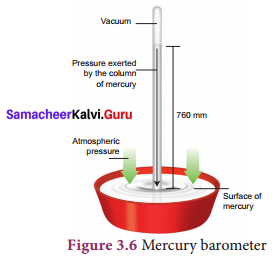The instrument used to measure atmospheric pressure is called barometer. A mercury barometer, first designed by an Italian Physicist Torricelli, consists of a long glass tube (closed at one end, open at the other) filled with mercury and turned upside down into a container of mercury. This is done by closing the open end of the mercury filled tube with the thumb and then opening it after immersing it in to a trough of mercury. The barometer works by balancing the mercury in the glass tube against the outside air pressure.

If the air pressure increases, it pushes more of the mercury up into the tub and if the air pressure decreases, more of the mercury drains from the tube. As there is no air trapped in the space between mercury and the closed end, there is vacuum in that space. Vacuum cannot exert any pressure. So the level of mercury in the tube provides a precise measure of air pressure which is called atmospheric pressure. This type of instrument can be used in a lab or weather station.

Class 9 Science Chapter 3 Question 3.
How does an object’s density determine whether the object will sink or float in water?
Whether an object will sink or float in a liquid is determined by the density of the object compared to the density of the liquid. If the density of a substance is less than the density of the liquid it will float. For example, a piece of wood which is less dense than water will float on it. Any substance having more density than water (for example, a stone), will sink into water.

Class 9 Science Chapter 3 Exercise Solutions Question 4.
Explain the construction and working of a hydrometer with diagram.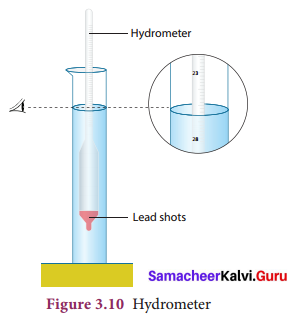A direct-reading instrument used for measuring the density or relative density of the liquid is called hydrometer. Hydrometer is based on the principle of flotation, i.e., the weight of the liquid displaced by the immersed portion of the hydrometer is equal to the weight of the hydrometer.

Hydrometer consists of a cylindrical stem having a spherical bulb at its lower end and a narrow tube at its upper end. The lower spherical bulb is partially filled with lead shots or mercury. This helps hydrometer to float or stand vertically in liquids. The narrow tube has markings so that relative density of a liquid can be read directly.

The liquid to be tested is poured into the glass jar. The hydrometer is gently lowered into the liquid until it floats freely. The reading against the level of liquid touching the tube gives the relative density of the liquid.

Class 9 Chapter 3 Science Question 5.
State the laws of flotation.
Laws of flotation are,

1. The weight of a floating body in a fluid is equal to the weight of the fluid displaced by the body.
2. The centre of gravity of the floating body and the centre of buoyancy are in the same vertical line.

VII. Assertion and Reason.

(a) If both assertion and reason are true and reason is the correct explanation of assertion.
(b) If both assertion and reason are true but reason is not the correct explanation of assertion.
(c ) If assertion is true but reason is false.
(d) If assertion is false but reason is true.

Chapter 3 Class 9 Science Pdf Question 1.
Assertion (A): To float, body must displace liquid whose weight is equal to the actual weight.
Reason (R): The body will experience no net downward force in that case.
(a) If both assertion and reason are true and reason is the correct explanation of assertion.

Class 9 Science Chapter 3 Exercise Solutions Question 2.
Assertion (A): Pascal’s law is the working principle of a hydraulic lift.
Reason (R): Pressure is thrust per unit area.
(b) If both assertion and reason are true but reason is not the correct explanation of assertion.

VIII. Numerical problems.

Question 1.
A block of wood of weight 200 g floats on the surface of water. If the volume of block is 300 cm3 calculate the upthrust due to water.
Upthrust of floating object = weight of the water displaced
Weight = mg
= 0.200Kg × $$\frac { 10m }{ s2 }$$
= 2N

Question 2.
Density of mercury is 13600 kg m– 3. Calculate the relative density.
Relative Density = $$\frac { Density of Mercury }{ Density of water }$$ at 4°C
R.D. = 13.6

Question 3.
The density of water is 1 g cm– 3. What is its density in S.I. units?
S.I. unit of density of water = $$\frac { 1000kg }{ m3 }$$

Question 4.
Calculate the apparent weight of wood floating on water if it weighs 100g in air.
Apparent weight = Weight of the body – Weight of liquid
Since the body is floating the two are equal. So, apparent weight is zero.
100 – 100 = 0

IX. HOTS

Question 1.
How high does the mercury barometer stand on a day when atmospheric pressure is 98.6 kPa?
H= $$\frac{P_{\max }}{P_{H_{g}}}$$ = 98.6 × 10 × 10 × 10
= $$\frac{(\mathrm{N} / \mathrm{m})^{2}}{13.6 \times 10 \times 10 \times 10 \mathrm{Kg} / \mathrm{m}^{3}}$$
= $$\frac { 9.8m }{ s2 }$$ = 740mm

Question 2.
How does a fish manage to rise up and move down in water?
Fish has an internal swim bladder which is filled with gas. When it needs to rise or descend, it changes the volume and its density by filling this bladder with oxygen collected from the surrounding water through gills. When the bladder is filled with oxygen gas, the fish has a greater volume, with minimal increase in weight. When the bladder is expanded, it displaces more water and so experiences a greater force of buoyancy.

When the bladder is completely inflated, the fish has maximum volume and is pushed to the surface. When the bladder is completely deflated, the fish has minimum volume and sinks to the ocean floor.

Question 3.
If you put one ice cube in a glass of water and another in a glass of alcohol, what would you observe? Explain your observations.
Ice floats in water and not in alcohol. This is because the density of ice is $$\frac { 0.917g}{ cc }$$ which is lower than that of water which is 1. Whereas, the density of ethanol (alcohol) is only $$\frac { 0.789g}{ cc }$$ which is lesser than ice, hence it floats in alcohol.

Question 4.
Why does a boat with a hole in the bottom would eventually sink?
A boat with a hole at the bottom allows water to enter it, thus increasing its weight and hence it sinks. As water starts entering the boat through the hole, the boat starts to get heavier, so it starts to sink, trying to displace more water. But the water keeps coming as the hydrostatic pressure at the hole is always higher than the atmospheric pressure pushing down on the surface of the water in the boat.

ACTIVITY

Question 1.
Take two identical flasks and fill one flask with water to 250 cm3 mark and the other with kerosene to the same 250 cm3 mark. Measure them in a balance. The flask filled with water will be heavier than the one filled with kerosene. Why? The answer is in finding the mass per unit volume of kerosene and water in respective flasks.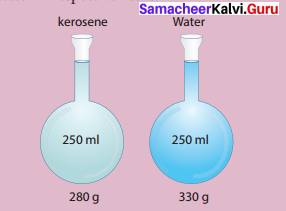To understand density better, let us assume that the mass of the flask be 80 g. So, the mass of the flask filled with water is 330 g and the mass of flask filled with kerosene is 280 g. Mass of water only is 250 g and kerosene only is 200 g.
Mass per unit volume of water = $$\frac { 250 }{ 250 }$$ cm3
= $$\frac { 1 g }{ cm3 }$$
Mass per unit volume of kerosene = 200 g/250cm3
= $$\frac { 0.8 g }{ cm3 }$$
The result $$\frac { 1g }{ cm3 }$$ and $$\frac { 0.8 g }{ cm3 }$$ are the densities of water and kerosene respectively.
Therefore the density of a substance is the mass per unit volume of a given substance.

### Samacheer Kalvi 9th Science Fluids In Text Problems

Question 1.
A man whose mass is 90 kg stands on his feet on a floor. The total area of contact of his two feet with the floor is 0.036 m2 (Take, g = 10 ms– 2). How much is the pressure exerted by him on the floor?
Solution:
The weight of the man (thrust),
F = mg = 90 kg × 10 ms– 2 = 900 N
Pressure, P = $$\frac{F}{A}=\frac{900 N}{0.036 m^{2}}$$ = 25000 Pa

Question 2.
Calculate the pressure exerted by a column of water of height 0.85 m (density of water, pw = 1000 kg m– 3) and kerosene of same height (density of kerosene, pk = 800 kg m– 3)
Solution:
Pressure due to water = hpwg = 0.85 m × 1000 kg m– 3 × 10 ms– 2 = 8500 Pa.
Pressure due to kerosene = hpkg = 0.85 m × 800 kg m– 3 × 10 ms– 2 = 6800 Pa.

Question 3.
A mercury barometer in a physics laboratory shows a 732 mm vertical column of mercury. Calculate the atmospheric pressure in pascal.
[Given density of mercury, p = 1.36 × 104kg m– 3, g = 9.8 ms– 2]
Solution:
Atmospheric pressure in the laboratory,
P = hpg = 732 × 10– 3 × 1.36 × 1o4 × 9.8
= 9.76 × 104 Pa (or) 0.976 × 105 Pa

Question 4.
A hydraulic system is used to lift a 2000 kg vehicle in an auto garage. If the vehicle sits on a piston of area 0.5 m2, and a force is applied to a piston of area 0.03 m2, what is the minimum force that must be applied to lift the vehicle?
Given: Area covered by the vehicle on the piston A1 = 0.5 m2
Weight of the vehicle, F1 = 2000 kg × 9.8 m s– 2
Area on which force F2 is applied, A2 = 0.03 m2
Solution:
P1 = P2 ;$$\frac{F_{1}}{A_{1}}=\frac{F_{2}}{A_{2}}$$and F2 = $$\frac{F_{1}}{A_{1}}$$A2 ;
F2 = (2000 × 9.8)$$\frac{0.03}{0.5}$$ = 1176 N

Question 5.
You have a block of a mystery material, 12 cm long, 11 cm wide and 3.5 cm thick. Its mass is 1155 grams.
(a) What is its density?
(b) Will it float in a tank of water, or sink?
Solution:
(a) Density = $$\frac{\text { Mass }}{\text { Volume }}=\frac{1155 \mathrm{g}}{12 \mathrm{cm} \times 11 \mathrm{cm} \times 3.5 \mathrm{cm}}=\frac{1155 \mathrm{g}}{462 \mathrm{cm}^{3}}$$ = 2.5g cm– 3
(b) The mystery material is denser than the water, so it sinks

### Samacheer Kalvi 9th Science Fluids Additional Questions

Question 1.
Give reasons why:
a. a single nail pricking the body is painful when compared to lying on a bed of nails.
b. cutting edges of knife and axes are sharpened.
c. heavy trucks are fitted with 6 to 8 wheels
a. In a nail bed, the weight is evenly distributed among numerous nails, so that the pressure exerted by each nail is not enough to puncture a person’s skin. If its only a single nail, the entire force created by the weight of the body would be distributed over a very small area presented by the tip of one nail. In this case the force per unit area will be great and can puncture the skin.
b. Knives and axes are sharpened because when the area decreases the pressure increases. Hence a small force is enough to cut an object.
c. As area increases pressure decreases. So weight of the truck exerts less pressure on the road.

Question 2.
Define:

1. Thrust,
2. Pressure

1. Force acting on a body perpendicular to the surface is called thrust.
2. The force per unit area acting on an object concerned is called pressure.

Question 3.
In petrol bunks, in what unit is tyre pressure measured?
The tyre pressure is measured in units of psi. it stands for pascal per inch.

Question 4.
Stating Pascal’s law, explain its application in a hydraulic press.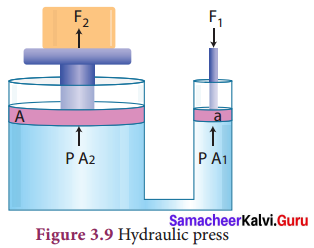Pascal’s law states that-the external pressure applied on an incompressible liquid is transmitted uniformly throughout the liquid. Pascal’s law became the basis for one of the important machines ever developed, the hydraulic press. It consists of two cylinders of different cross-sectional areas. They are fitted with pistons of cross-sectional areas “a” and “A”. The object to be compressed is placed over the piston of large cross-sectional area A. The force Fj is applied on the piston of small cross-sectional area a.

The pressure P produced by small piston is transmitted equally to large piston and a force F2 acts on A which is much larger than F1 area ‘a’ is given by,
P = $$\frac { F1}{ A1}$$ …………………….(1)
Applying Pascal’s law, the pressure on large piston of area A will be the same as that on small piston. Therefore, P = $$\frac { F2 }{ A2 }$$ …………… (2)
Comparing equations (1) and (2),we get
$$\frac { F1 }{ A1 }$$ = $$\frac { F2 }{ A2 }$$ or F2 = F1 × $$\frac { A2 }{ A1 }$$
Since, the ratio $$\frac { A2 }{ A1 }$$ is greater than 1, the force F2 that acts on the larger piston is greater than the force F1 acting on the smaller piston. Hydraulic systems working in this way are known as force multipliers.

Question 5.
What is Artisan aquifer?
An artesian aquifer is a confined aquifer (an underground water bearing permeable rocks) containing groundwater that will flow upwards out of a well without the need for pumping. In recharging aquifers, this happens because the water table at its recharge zone is at a higher elevation than the head of the well.

Question 6.
What is relative density? Explain it mathematically.
Density of any other substance with respect to the density of water at 4°C is called relative density. Mathematically,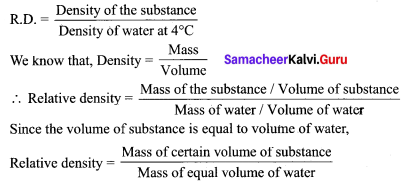Thus, the ratio of mass of a given volume of a substance to the mass of an equal volume of water at 4°C also denotes relative density.

Question 7.
What is lactometer? Explain its principle and working.
One form of hydrometer is a lactometer, an instrument used to check the purity of milk. The lactometer works on the principle of gravity of milk. The lactometer consists of a long graduated test tube with a cylindrical bulb with the graduation ranging from 15 at the top to 45 at the bottom. The test tube is filled with air. This air chamber causes the instrument to float. The spherical bulb is filled with mercury to cause the lactometer to sink up to the proper level and to float in an upright position in the milk.

Inside the lactometer there may be a thermometer extending from the bulb up into the upper part of the test tube where the scale is located. The correct lactometer reading is obtained only at the temperature of 60°C. A lactometer measures the cream content of milk.

More the cream, lower the lactometer floats in the milk. The average reading of normal milk is 32. The lactometers are used highly at milk processing units and at dairies.

Question 8.
Why do petroleum based products float on surface of water?
Petroleum based products float on water because their specific gravity is low.

Question 9.
State and explain Archimedes principle. Name the devices based on this principle.